# Data Structures — ArrayNow, We will start talking about Array as the simplest data structure that we can deal with.

Array is used to store similar type data.

We need array in cases we have a large number of data from the same type and we want to store them in memory. in normal case we will need a large number of variables to store this data.

But Array will help us to store all the data in just one variable.

Array elements stored in sequence of memory locations and the first element in the array will be stored in the lowest memory location and will be called base address.

In most programing language, array is zero-index which means that if we have array with n elements, the first element will be in index 0 and second will be in index 1….until the last element will be in index n-1.

#### How to declare array?

type array_name[size];

example:

int arr===> this “arr” array with size 3 that will store data of type integer.

#### How to access elements inside array?

We can access the elements inside the array by its index.

example:

arr==> we want to access second element, because “arr” is zero index array.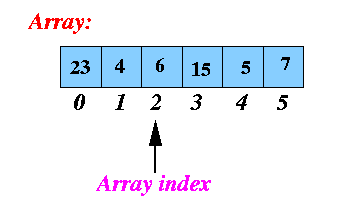The following java code can explain how to set data in array and how to restore them to use them later.

```private static void setArrayElements(int[] arr, int n)
{
for(int i=0;i<n;i++){
arr[i] = i+5;
}
}

private static void printElemnt(int[] arr, int n)
{
for(int i=0;i<n;i++){
System.out.printf(“element index = %d, element value =%d\n”,i,arr[i]);
}
}
```

2D Array:-

Two Dimensional array is can be defined as array of array which means that 2D array is something like matrix and data will be sorted in rows and columns.

2D array is a human representation for database tables.

How to declare 2D array?

type array_name[rows size][columns size]; example: int arr===> this “arr” array with 3 rows and 3 columns where will store data of type integer.

#### How to access elements inside array?

We can access the elements inside the array by its row index and column index. example: arr==> we want to access an element in the first row and second column because rows of the array and columns of the array are 0-index. the following picture can explain the 2D array more:-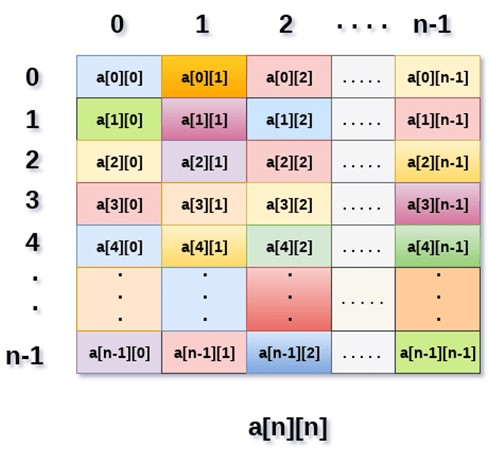We usually need to map the 2D array into 1D array, and that can be done based on rows or columns.

#### Mapping based on rows:-

In this mapping we will take the elements of first row, then second ,…, to the n-1 row and store them in 1D array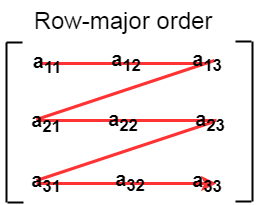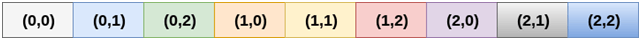#### Mapping based on columns:-

In this mapping we will take the elements of first column, then second ,…, to the n-1 column and store them in 1D array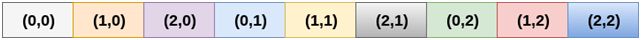and here is a code that can explain how to set data in 2D array and how to use them after that:-

```private static void setArrayElements(int[][] arr,int r, int n)
{
for(int i=0;i<r;i++){
for(int j=0;j<n;j++){
arr[i][j] = j*i+5;
}
}
}
private static void printElemnt(int[][] arr, int r, int n)
{
for(int i=0;i<r;i++){
for(int j=0;j<n;j++){
System.out.printf(“element row index = %d, element column index = %d , element value =%d\n”,i,j,arr[i][j]);
}
}
}
```

Feel free to check this git repository to know how to implement and use 1D and 2D arrays.Heba Tarek Heba is a software engineer with five years of experience in the complete software development life cycle with extensive knowledge in PHP, Ruby on Rails, Java SE, MySQL, Restful, Web Services. She is a Professional Scrum Master certified by scrum.org